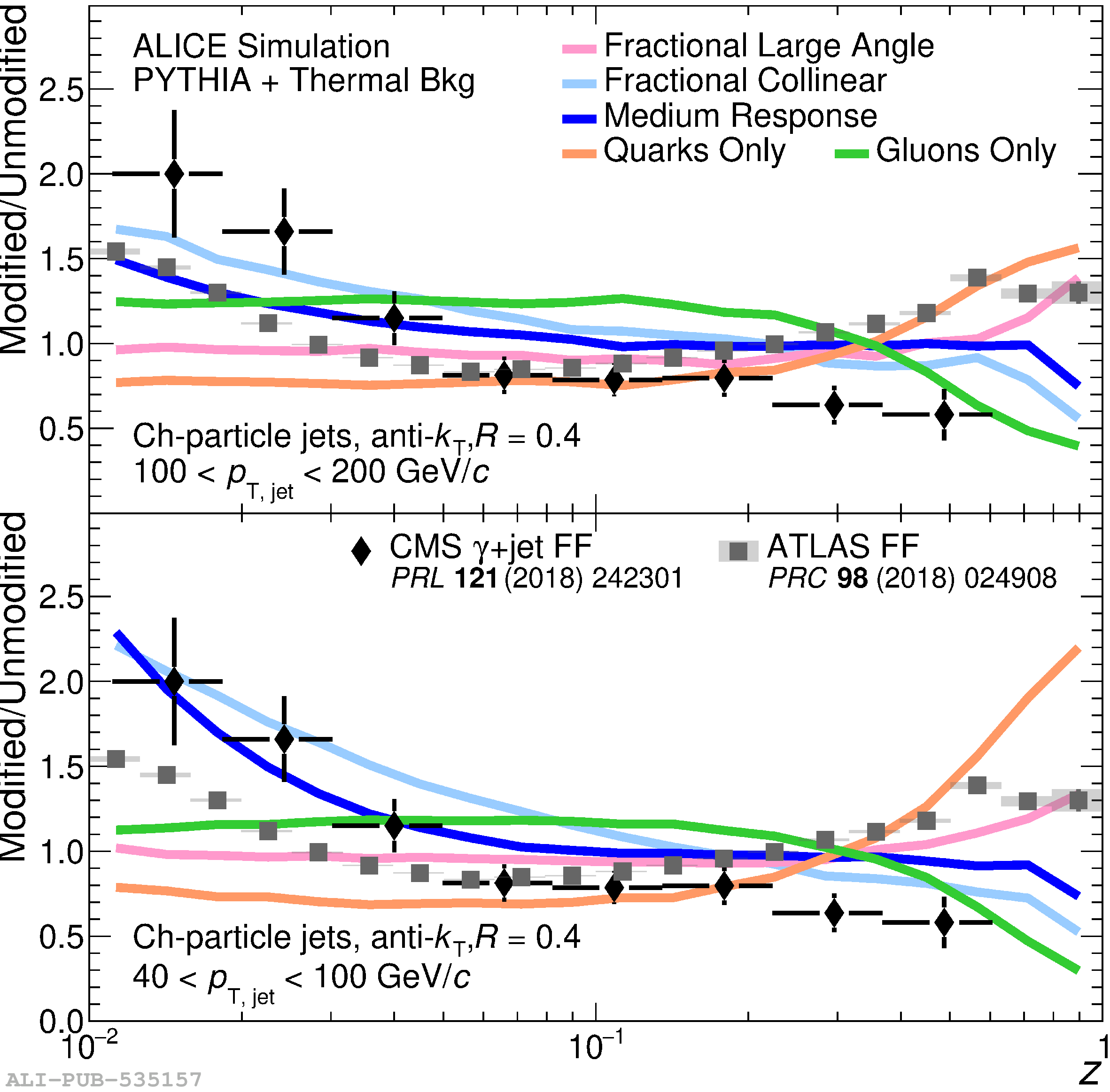# Figure 2

 Left: Comparison of toy model modifications for $R = 0.4$ jets using the $R^{\rm toy}_{\rm AA}$ as defined in Eq. (4). Right: The fragmentation functions at low jet $p_{\rm T}$ ($40 < p_{\rm T, true} < 100 \text{ GeV}/c$, lower right panel) and high jet $p_{\rm T}$ ($100 < p_{\rm T, true} < 200 \text{ GeV}/c$, upper right panel) for 0$-$10% central Pb$-$Pb collisions. In the fractional collinear and fractional large angle case, $f_{\rm loss} = 25\%$ and $p_{\rm loss} = 100\%$. In the medium response case, $f_{\rm loss} = 10\%$ and $p_{\rm loss} = 50\%$ The ratio of the fragmentation functions measured in Pb$-$Pb and pp collisions are shown for jets with $R=0.4$ and $\pt>100$ GeV/$c$  (ATLAS), and for jets with $R=0.3$ and $p_{\rm T} > 30$ GeV/$c$ recoiling from a photon with $E_{\rm T}>60$ GeV/$c$  (CMS).